Courses

# Test: Patterns

## 10 Questions MCQ Test Mathematics for Class 3 | Test: Patterns

Description
This mock test of Test: Patterns for Class 3 helps you for every Class 3 entrance exam. This contains 10 Multiple Choice Questions for Class 3 Test: Patterns (mcq) to study with solutions a complete question bank. The solved questions answers in this Test: Patterns quiz give you a good mix of easy questions and tough questions. Class 3 students definitely take this Test: Patterns exercise for a better result in the exam. You can find other Test: Patterns extra questions, long questions & short questions for Class 3 on EduRev as well by searching above.
QUESTION: 1

### What shape are the faces of a cube?

Solution:

Each face of a cube is shaped like a square. The sides of each face are called edges. A cube has 12 edges.

QUESTION: 2

### Which of the following is the BEST example of a sphere?

Solution:

A sphere is a geometrical object in three-dimensional space that is the surface of a ball. Like a circle in a two-dimensional space, a sphere is defined mathematically as the set of points that are all at the same distance r from a given point in a three-dimensional space.
Examples of sphere include: Ball. Planets. Moon.

QUESTION: 3

### Look at the pairs of shapes. Which shows a pair of rectangle?

Solution:

Option D shows a pair of a rectangle because :
⇒ The figures has four sides.
⇒ Opposite sides are parallel, congruent and equal.
⇒ And all four angles are 90
⇒ So, option D justify the all properties of rectangle and other options don't then option D is a right option.

QUESTION: 4

Which shape has fewer than four sides?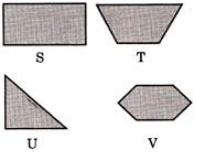Solution: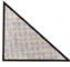It has three sides.

QUESTION: 5

How many edges does the following figure have?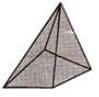Solution:

By observing the figure it can be clearly said that the following figure have 8 edges.

QUESTION: 6

Which of the following figure has six faces?

Solution:

Cube has 6 faces

QUESTION: 7

Which of the following figure contains exactly three circles?

Solution:

Option C. The figure contains exactly three circles.

QUESTION: 8

Which of the following is different from other three?

Solution:

Only 'D' is closed figure.

QUESTION: 9

Which of the following is different from the other three?

Solution:

All other are made up of lines.

QUESTION: 10

Which shape is divided into two EQUAL parts by the dotted line?

Solution:

The dotted line  divides the figure of option D in  2 equal parts, while the other options are not divided symmetrically
∴ Correct answer is option D.

Track your progress, build streaks, highlight & save important lessons and more!

### Similar Content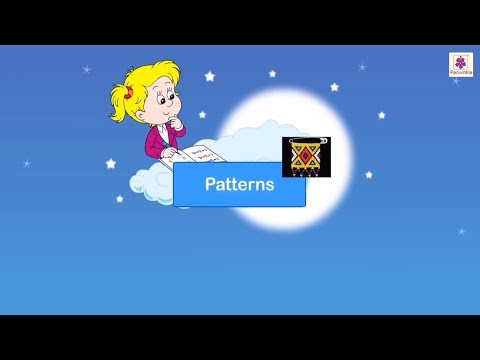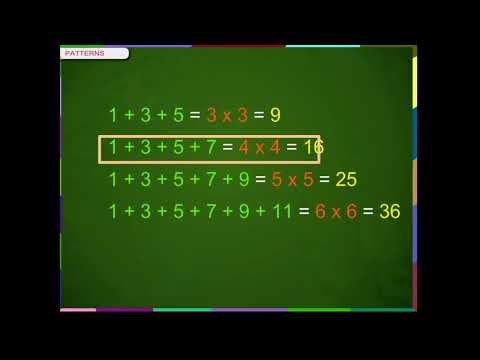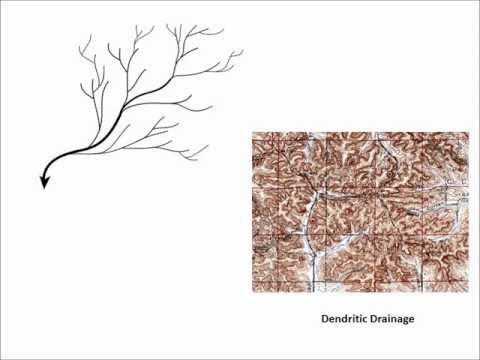### Related tests